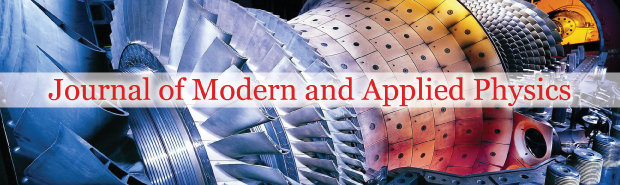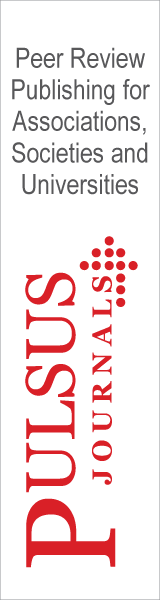All submissions of the EM system will be redirected to Online Manuscript Submission System. Authors are requested to submit articles directly to Online Manuscript Submission System of respective journal.### Euro Physics 2018 - Measurement Induced Nonlocality Based On Fidelity -Muthuganesan Rajendra - National Institute of Technology, Tiruchirappalli.

Author(s): Muthuganesan Rajendra

Quantum correlation, a fundamental aspect of quantum mechanics and significantly makes the departure from the classical regime. It is a useful physical resource for various quantum information processing such as teleportation, super dense coding, communication, and quantum algorithm. Quantification of correlation between its local constituents of a system is a formidable task in the framework of quantum information theory. In this regard, entanglement is believed as a valid resource for quantum advantageous in the early 19th century. In the light of seminal work of Werner  and the presence of non-zero quantum correlation namely, discord, it is believed that entanglement is alone not responsible for the advantageous over the classical algorithm. Recently, various measures have been proposed to capture quantum-ness, which goes beyond entanglement such as measurement-induced disturbance (MID), geometric discord (GD), measurement-induced nonlocality (MIN)  and uncertainty-induced nonlocality (UIN) . The local disturbance is an important tool to probe nonlocal aspects of a quantum state. In particular, locally invariant von Neumann projective measurements can induce global or nonlocal effects. Using local projectors of marginal states various measures have been studied extensively using local eigen projectors. Distance between the quantum state in state space is useful in quantification of quantum correlation. Geometric measures are easy to compute and experimentally realizable. In this context, several distance measures that have been introduced the, few such measures are trace distance, Hilbert-Schmidt norm, Jensen-Shannon divergence, fidelity induced metric and Hellinger distance. Recently, measurement based non-classical correlations have been characterized using various distance measures. MIN, characterize the non-locality of a quantum state in the perspective of locally invariant projective measurements, is more general than the Bell nonlocality. It is also a bona fide measure of quantum correlation for the bipartite state. MIN has also been investigated based on relative entropy, von Neumann entropy, skew information, trace distance and fidelity. In this article, we introduce a new variant of MIN based on affinity. It is shown that this quantity is a remedy for the local ancillary problem of Hilbert-Schmidt norm based MIN. This measure satisfies all criteria of a bona fide measure of quantum correlation 2 of bipartite system. Affinity–based MIN is a valid resource for quantum communication, cryptography and dense coding. Further, we evaluate the affinity–based MIN analytically for arbitrary pure state. We obtain the upper bound for m × n dimensional mixed state and a closed formula of the proposed MIN for the 2 × n dimensional mixed state. We study the quantumnes’s of well-known family of two-qubit m × m dimensional mixed state’s. Finally, we study the dynamical behaviour of affinity based measure under a noisy quantum channel.

MEASUREMENT-INDUCED NONLOCALITY

Measurement-induced nonlocality, captures nonlocal or global effect of a quantum state due to von Neumann projective measurements, is originally defined as maximal square of Hilbert-Schmidt norm of the difference of pre- and post- measurement states. Mathematically, it is defined as

N(ρ) = max ΠA kρ − Π A (ρ)k 2

Here the maximum is taken over the von Neumann projective measurements on subsystem A, ΠA(ρ) = P k (ΠA k 1 B)ρ(ΠA k 1 B), and ΠA = {ΠA k } = {|kihk|} being the projective measurements on the subsystem A, which do not change the marginal state ρ A locally i.e., ΠA(ρ A) = ρ A. The dual of this quantity is geometric discord (GD) of the given state ρ formulated as

D(ρ) = min ΠA kρ − Π A (ρ)k 2

For nondegenerate ρ A the optimization is not required. Hence, both the MIN and GD are equal. Due to the computability and easy accessibility of experimentation, the researchers paid much attention to the both GD and MIN. However, both the quantities are having an unwanted property of quantum correlation measure, it may change rather arbitrarily through some trivial and uncorrelated action on the unmeasured party B.                                                                                                kXk → kΓ σXk = kXk √ Trσ

After the addition of local ancilla ρ C, MIN of the resultant state is computed as

N(ρ A:BC) = N(ρ AB)Tr(ρ C ) 2

Implying that MIN differs arbitrarily due to local ancilla C as long as ρ C is mixed. Since the optimization over the projective measurements on A is unaffected by the presence of uncorrelated ancilla state on B (unmeasured party). Thus, adding or removing local ancilla – a local and reversible operation. Due to this operation, a factor was namely, purity of the ancillary state is added to the original MIN. A natural way to resolve this local ancilla problem is to redefine the MIN (1) as

N˜(ρ) = max ΛB N(ρ AB)ΛB

Where the maximum is over the channel ΛB. On the other way, Luo and Fu remedied this local ancilla problem by replacing density matrix by its square root i.e., mathematically remedied MIN is defined as

N(ρ) = max ΠA k √ ρ − Π A ( √ ρ)k 2 .

Further, the contractive distance measures are such as the trace distance, Hellinger distance and fidelity induced metric are also useful in resolve this local ancillary problem. In what follows, we define a new variant of MIN using affinity induced metric.

CONCLUSIONS

In this article, we have proposed a new variant of measurement induced nonlocality (MIN) using affinity metric as a measure of quantum correlation for bipartite state. It is shown that, in addition to capturing the global non-local effect of a state due to von Neumann projective measurements, this quantity can be remedying local ancilla problem of Hilbert– Schmidt norm based MIN. We have presented a closed formula of affinity–based MIN for an arbitrary 2 × n dimensional mixed state, with an upper bound for m × n dimensional system. Further, we have computed the proposed version of MIN for m × m dimensional Werner and iso-tropic states. Finally, we have studied the dynamics of MINs and compared with entanglement. It is shown that MINs are more robust against decoherence than the entanglement.

PDF

###### Journal of Modern and Applied Physics peer review process verified at publonsTop